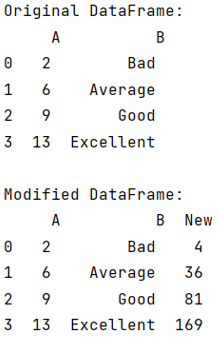# Square of each element of a column in pandas

Given a pandas dataframe, we have to find the square of each element of a column.
Submitted by Pranit Sharma, on September 12, 2022

Pandas is a special tool that allows us to perform complex manipulations of data effectively and efficiently. Inside pandas, we mostly deal with a dataset in the form of DataFrame. DataFrames are 2-dimensional data structures in pandas. DataFrames consist of rows, columns, and data.

## Finding the square of each element of a column in pandas

Suppose, we are given a DataFrame, we need to square each element of a column and store it in another new column.

Columns are the different fields that contain their particular values when we create a DataFrame. We can perform certain operations on both rows & column values. Each column has a specific header/name.

For this purpose, we will first create a new column by using the following syntax:

```df['new'] =
```

Here, after the = sign, we will write our expression whose result will be stored in the new column hence, we will compute the square of each value of a particular column and store it in the new column.

Let us understand with the help of an example,

## Python code to find the square of each element of a column

```# Importing pandas package
import pandas as pd

# Creating a dictionary
d = {
'A':[2,6,9,13],
}

# Creating DataFrame
df = pd.DataFrame(d)

# Display the DataFrame
print("Original DataFrame:\n",df,"\n")

# Creating new column and computing square
df['New'] = df['A']**2

# Display modified DataFrame
print("Modified DataFrame:\n",df,"\n")
```

Output:What's New (MCQs)

Top Interview Coding Problems/Challenges!

Languages: » C » C++ » C++ STL » Java » Data Structure » C#.Net » Android » Kotlin » SQL
Web Technologies: » PHP » Python » JavaScript » CSS » Ajax » Node.js » Web programming/HTML
Solved programs: » C » C++ » DS » Java » C#
Aptitude que. & ans.: » C » C++ » Java » DBMS
Interview que. & ans.: » C » Embedded C » Java » SEO » HR
CS Subjects: » CS Basics » O.S. » Networks » DBMS » Embedded Systems » Cloud Computing
» Machine learning » CS Organizations » Linux » DOS
More: » Articles » Puzzles » News/Updates

© https://www.includehelp.com some rights reserved.